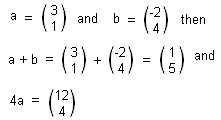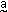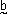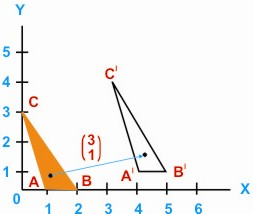HOME MATH DICTIONARY DOWNLOAD FEEDBACK DISCLAIMER
 Question: What is Vector ? Answer: Ordered sets of numbers which can be added by adding the numbers in corresponding positions, and which can be multiplied by a constant, are known as vectors. For example, ifIn print vectors are usually labeled with letters in bold type as shown but when writing it is usual to put a squiggle under any letter representing a vector such asand. The number of elements in a vector corresponds to the dimension of its space, so the examples shown are 3-dimensional. Vectors can be used to represent changes of positions and translations. In the diagram, the triangle ABC has been translated to triangle A'B'C'. Each point of the triangle has been displaced 3 units to the right and 1 unit up. An arrow such as PP' is often used on a diagram to represent the displacement vector.Quantities such as acceleration, velocity, force, displacement and momentum, which have direction as well as magnitude and can be combined by vector addition, are called vector quantities. In contrast quantities such as distance, energy and speed, which have magnitude but no direction, are called scalar quantities.